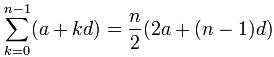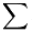# Arithmetic Sequences and Sums

## Sequence

A Sequence is a set of things (usually numbers) that are in order.Each number in the sequence is called a term (or sometimes "element" or "member"), read Sequences and Series for more details.

## Arithmetic Sequence

In an Arithmetic Sequence the difference between one term and the next is a constant.

In other words, we just add the same value each time ... infinitely.

### Example:

 1, 4, 7, 10, 13, 16, 19, 22, 25, ...

This sequence has a difference of 3 between each number.
The pattern is continued by adding 3 to the last number each time, like this:In General we could write an arithmetic sequence like this:

{a, a+d, a+2d, a+3d, ... }

where:

• a is the first term, and
• d is the difference between the terms (called the "common difference")

### Example: (continued)

 1, 4, 7, 10, 13, 16, 19, 22, 25, ...

Has:

• a = 1 (the first term)
• d = 3 (the "common difference" between terms)

And we get:

{a, a+d, a+2d, a+3d, ... }

{1, 1+3, 1+2×3, 1+3×3, ... }

{1, 4, 7, 10, ... }

### Rule

We can write an Arithmetic Sequence as a rule:

xn = a + d(n−1)

(We use "n−1" because d is not used in the 1st term).

### Example: Write a rule, and calculate the 9th term, for this Arithmetic Sequence:

 3, 8, 13, 18, 23, 28, 33, 38, ...

This sequence has a difference of 5 between each number.The values of a and d are:

• a = 3 (the first term)
• d = 5 (the "common difference")

Using the Arithmetic Sequence rule:

xn = a + d(n−1)

= 3 + 5(n−1)

= 3 + 5n − 5

= 5n − 2

So the 9th term is:

x9 = 5×9 − 2
= 43

Is that right? Check for yourself!

Arithmetic Sequences are sometimes called Arithmetic Progressions (A.P.’s)

## Advanced Topic: Summing an Arithmetic Series

To sum up the terms of this arithmetic sequence:

a + (a+d) + (a+2d) + (a+3d) + ...

use this formula:What is that funny symbol? It is called Sigma Notation(called Sigma) means "sum up"

And below and above it are shown the starting and ending values:It says "Sum up n where n goes from 1 to 4. Answer=10

Here is how to use it:

### Example: Add up the first 10 terms of the arithmetic sequence:

{ 1, 4, 7, 10, 13, ... }

The values of a, d and n are:

• a = 1 (the first term)
• d = 3 (the "common difference" between terms)
• n = 10 (how many terms to add up)

So:Becomes:= 5(2+9·3) = 5(29) = 145

Check: why don't you add up the terms yourself, and see if it comes to 145

## Footnote: Why Does the Formula Work?

Let's see why the formula works, because we get to use an interesting "trick" which is worth knowing.

First, we will call the whole sum "S":

S = a + (a + d) + ... + (a + (n−2)d) + (a + (n−1)d)

Next, rewrite S in reverse order:

S = (a + (n−1)d) + (a + (n−2)d) + ... + (a + d) + a

Now add those two, term by term:

 S = a + (a+d) + ... + (a + (n-2)d) + (a + (n-1)d) S = (a + (n-1)d) + (a + (n-2)d) + ... + (a + d) + a 2S = (2a + (n-1)d) + (2a + (n-1)d) + ... + (2a + (n-1)d) + (2a + (n-1)d)

Each term is the same! And there are "n" of them so ...

2S = n × (2a + (n−1)d)

Now, just divide by 2 and we get:

S = (n/2) × (2a + (n−1)d)

Which is our formula: